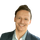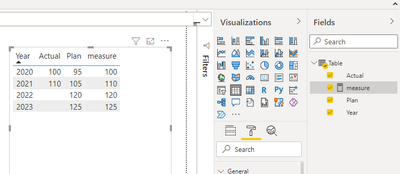cancel
Showing results for
Did you mean:## Combine actual and target based on date

I want to create a simple measure but I fail to get it.

There are actual and plan values. I want to create a measure that sums actual values as soon as there are any values. If there is no actual value what so over the measure should use the plan value.I have tried to write a measure but it is not working.

``````New Measure =
var actual_values_maxDate =
CALCULATE(
MAX( 'Table'[Date] ),
ALL( 'Table' ),
'Table'[Planning Type] = "actual"
)

RETURN
IF(
//Logical Test
SELECTEDVALUE( 'Date'[Date] ) > actual_values_maxDate,
//if true
CALCULATE(
SUM( 'Table'[Value] ),
FILTER( 'Table', 'Table'[Planning Type] = "plan" )
),
//if false
CALCULATE(
SUM( 'Table'[Value] ),
FILTER( 'Table', 'Table'[Planning Type] = "actual" )
)
)``````

1 ACCEPTED SOLUTIONCommunity Support

Based on your example, you can try this measure,

``````measure =
var _actual= MIN('Table'[Actual])
var _plan= MIN('Table'[Plan])
return IF(ISBLANK(_actual),_plan,_actual)``````Best Regards,

Community Support Team _Tang

If this post helps, please consider Accept it as the solution to help the other members find it more quickly.

4 REPLIES 4Community Support

Based on your example, you can try this measure,

``````measure =
var _actual= MIN('Table'[Actual])
var _plan= MIN('Table'[Plan])
return IF(ISBLANK(_actual),_plan,_actual)``````Best Regards,

Community Support Team _Tang

If this post helps, please consider Accept it as the solution to help the other members find it more quickly.@v-xiaotang Thank you. That seemed to work.

I have adjusted the formular a little bit according to my case

``````FTE =
var _actual= CALCULATE( SUM('Internal'[FTE] ),'Internal'[Planning Type] = "actual" )
var _plan= CALCULATE( SUM('Internal'[FTE] ),'Internal'[Planning Type] = "plan" )
RETURN
IF(
//logical test
ISBLANK(_actual),
//if true
_plan,
//if false
_actual
)``````Super User

@raymondpocher , Try with small change

New Measure =
var actual_values_maxDate =
CALCULATE(
MAX( 'Table'[Date] ),
Filter( ALL( 'Table' ),
'Table'[Planning Type] = "actual" && not(isblank('Table'[Value] )) )
)

RETURN
Sumx( values(Table[Year]), IF(
//Logical Test
Max( 'Date'[Date] ) > actual_values_maxDate,
//if true
CALCULATE(
SUM( 'Table'[Value] ),
FILTER( 'Table', 'Table'[Planning Type] = "plan" )
),
//if false
CALCULATE(
SUM( 'Table'[Value] ),
FILTER( 'Table', 'Table'[Planning Type] = "actual" )
)
) )@amitchandak  I dont know why but for some reason I wasnt able to manage it with that formular.